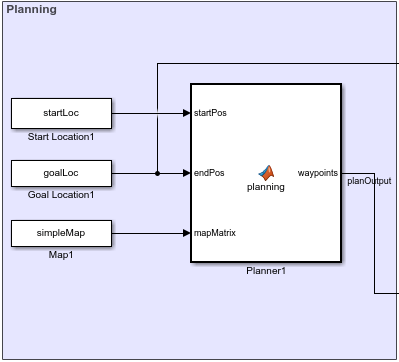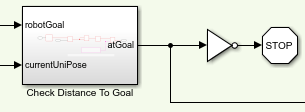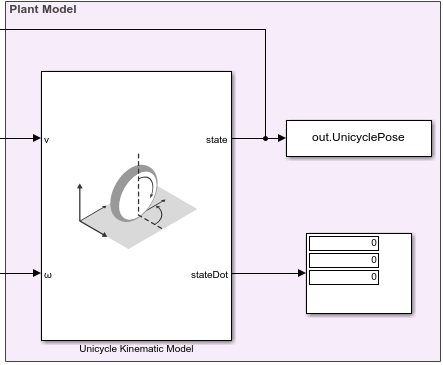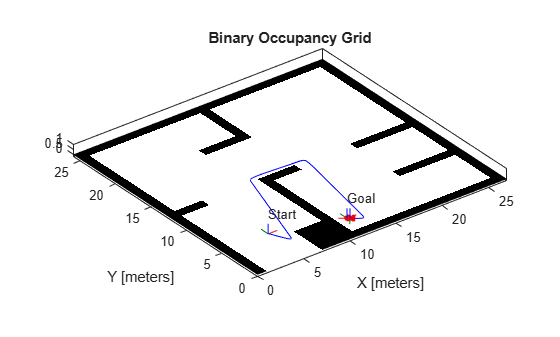# Plan Path for a Unicycle Robot in Simulink

This example demonstrates how to execute an obstacle-free path between two locations on a given map in Simulink®. The path is generated using a probabilistic road map (PRM) planning algorithm (`mobileRobotPRM)`. Control commands for navigating this path are generated using the Pure Pursuit controller block. A unicycle kinematic motion model simulates the robot motion based on those commands.

Load the occupancy map, which defines the map limits and obstacles within the map. `exampleMaps.mat` contain multiple maps including `simpleMap`, which this example uses.

`load exampleMaps.mat`

Specify a start and end location within the map.

```startLoc = [5 5]; goalLoc = [12 3];```

### Model Overview

`open_system('pathPlanningUnicycleSimulinkModel.slx')`

The model is composed of three primary parts:

• Planning

• Control

• Plant Model

#### PlanningThe Planner MATLAB® function block uses the `mobileRobotPRM` path planner and takes a start location, goal location, and map as inputs. The blocks outputs an array of waypoints that the robot follows. The planned waypoints are used downstream by the Pure Pursuit controller block.

#### Pure PursuitThe Pure Pursuit controller block generates the linear velocity and angular velocity commands based on the waypoints and the current pose of the robot.

#### Check if Goal is ReachedThe Check Distance to Goal subsystem calculates the current distance to the goal and if it is within a threshold, the simulation stops.

#### Plant ModelThe Unicycle Kinematic Model block creates a vehicle model to simulate simplified vehicle kinematics. The block takes linear and angular velocities as command inputs from the Pure Pursuit controller block, and outputs the current position and velocity states.

### Run the Model

To simulate the model

`simulation = sim('pathPlanningUnicycleSimulinkModel.slx');`

### Visualize The Motion of Robot

After simulating the model, visualize the robot driving the obstacle-free path in the map.

`map = binaryOccupancyMap(simpleMap)`
```map = binaryOccupancyMap with properties: mapLayer Properties LayerName: 'binaryLayer' DataType: 'logical' DefaultValue: 0 GridLocationInWorld: [0 0] GridOriginInLocal: [0 0] LocalOriginInWorld: [0 0] Resolution: 1 GridSize: [26 27] XLocalLimits: [0 27] YLocalLimits: [0 26] XWorldLimits: [0 27] YWorldLimits: [0 26] ```
`robotPose = simulation.UnicyclePose`
```robotPose = 428×3 5.0000 5.0000 0 5.0000 5.0000 -0.0002 5.0001 5.0000 -0.0012 5.0006 5.0000 -0.0062 5.0031 5.0000 -0.0313 5.0156 4.9988 -0.1569 5.0707 4.9707 -0.7849 5.0945 4.9354 -1.1140 5.1075 4.9059 -1.1828 5.1193 4.8759 -1.2030 ⋮ ```
```numRobots = size(robotPose, 2) / 3; thetaIdx = 3; % Translation xyz = robotPose; xyz(:, thetaIdx) = 0; % Rotation in XYZ euler angles theta = robotPose(:,thetaIdx); thetaEuler = zeros(size(robotPose, 1), 3 * size(theta, 2)); thetaEuler(:, end) = theta; for k = 1:size(xyz, 1) show(map) hold on; % Plot Start Location plotTransforms([startLoc, 0], eul2quat([0, 0, 0])) text(startLoc(1), startLoc(2), 2, 'Start'); % Plot Goal Location plotTransforms([goalLoc, 0], eul2quat([0, 0, 0])) text(goalLoc(1), goalLoc(2), 2, 'Goal'); % Plot Robot's XY locations plot(robotPose(:, 1), robotPose(:, 2), '-b') % Plot Robot's pose as it traverses the path quat = eul2quat(thetaEuler(k, :), 'xyz'); plotTransforms(xyz(k,:), quat, 'MeshFilePath',... 'groundvehicle.stl'); pause(0.01) hold off; end```